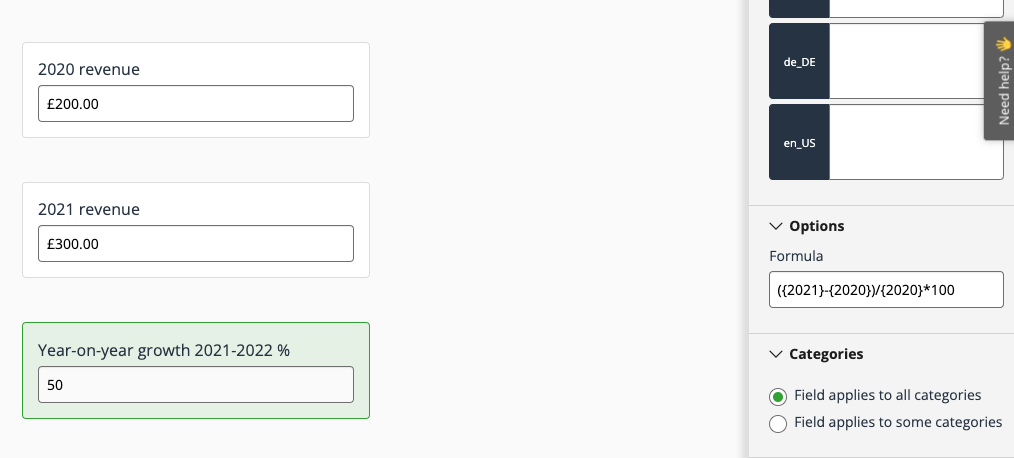# Utilising formula fields

The formula field instantly performs calculations based on values added to other fields on the entry form. To create your formula you'll need to reference the slugs or short titles of these fields. This must be enclosed in curly braces.

Note: all field types are supported excluding table fields, content fields, and other formula fields.

## Configure a formula field

1. In the Manage workspace, navigate to Entries and click Edit form
2. Locate where you'd like to place your formula field click the + that appears
3. In the configuration tray on the right side of the page, select Formula from the Field type drop-down
4. Provide a Label and Short title
5. If desired, add in Hint and/or Help text to provide context to entrants
6. In the 'Options' section, key in your formula utilising the functions listed below
7. Under the 'Access' heading, choose whether this field is hidden from entrants by deselecting the Read checkbox if necessary
Tip: hidden fields can be shown to judges during the evaluation process, for more information see: Show/hide fields for judging
8. Save when finished

## Examples

If you want to calculate the year-on-year growth in revenue for two fields with short titles "2020" and "2021" you would reference each field as {2020} and {2021} in your formula. Here's how it will look:

`({2021}-{2020})/{2020}*100`To round the result to two decimal places you can use the round function as follows:

`round(({2021}-{2020})/{2020}*100,2)`

## Available functions

The formula field supports a number of different functions, many of which will be familiar if you use formulas in Excel and other spreadsheet tools. Here are some examples of what you can do.

### Number and currency fields

 AverageTakes the average of the specified fields average({field1},{field2},{field3},{field4}) SumSums the specified fields sum({field1},{field2},{field3},{field4}) MinReturns the smallest number of the specified fields min({field1},{field2},{field3},{field4}) MaxReturns the largest number of the specified fields max({field1},{field2},{field3},{field4}) RoundRounds to specified number of decimal places round(number,count) Round upRounds up to specified number of decimal places roundup(number,count) Round downRounds down to specified number of decimal places rounddown(number,count)

### Strings

 ConcatenateCombines several text strings into one string concatenate({field1},{field2},...{fieldn}) ExactReturns true if both strings are exactly the same day({field1},{field2}) LeftExtracts a given number of characters from the left side of a text string. left({field},4) LowerConverts the text to lowercase lower({field}) MidReturns a substring of a given length starting from a specific position mid({field},start_position,length) ProperCapitalises the first word of a string proper({field}) ReplaceReplaces a substring of a given string replace({field},start_position,length,"new string") RightExtracts a given number of characters from the right side of a text string. right({field},4)

### Dates

 TodayReturns today's date today() To format the date use text(today(),"MM/DD/YYYY") DaysCalculates the difference between two date values days({field1},{field2}) MonthReturns the month for a date value month({field1}) YearReturns the year for a date value year({field1}) DayReturns the day for a date value day({field1},{field2}) EdateShifts the date by the given number of months edate({date},months)To format the date use text(edate({start},3),"dd/mm/yyyy") HourReturns the hour for a given time hour({time}) NowThe current date and time now()To format use:text(now(),"dd/mm/yy hh:mm:ss") WeekdayReturns the number that corresponds to a day of the week weekday({field}) Week numberReturns the number that corresponds to the week of the year weeknum({field}) Age Returns the age for a given date rounddown(days(today(),({DOB}))/365,0) Countdown Calculates the days and hours until a given date and time days({Date 2}, {Date 1}) & " days, " & hour({Date 2}) - hour({Date 1}) & " hours"

### Engineering

 bin2decConverts a binary number to decimal bin2dec({field}) bin2hexConverts a binary number to hexadecimal bin2hex({field}) dec2binConverts a decimal number to binary dec2bin({field}) dec2hexConverts a decimal number to hexadecimal dec2hex({field}) deltaReturns true(1) if both numbers are equal otherwise false(0) delta({field1},{field2})

### Information

 isnumberReturns true if the field contains a number isnumber({field1}) isevenReturns true if the field contains an even number iseven({field}) isoddReturns true if the field contains an odd number isodd({field}) istextReturns true if the field contains text istext({field})

### Logical

 ifIf/then test if({field1}>1000,"then value1", "otherwise value2") andReturns true if all arguments are true and({num1}=3, {num2}=3) orReturns true if at least one argument is true or({num1}=3, {num2}=3)

## Good to know

• Unlike Excel or many other spreadsheet tools, it isn't necessary to add a = symbol to indicate the start of a formula.
• The formula is stored referencing the field slugs, not the short title. If a field's short title is changed later, existing formulas that reference that field will not break.
• Calculated values are stored on the entry record when the entry is saved. This will allow a formula field column to be added to the many of the views in the platform, such as Entries in the Manage workspace.
• Changes to a formula after entries already exist with saved values will trigger a recalculation of all stored values.

To learn about other field types supported by Award Force, check out our guide: Field types overview.

## Need more help? Get in touch!

Simply send us a message via this form and we will get back to you quickly!
Our globally distributed client success team are here to help 24 hours a day, Monday to Friday (business hours).